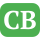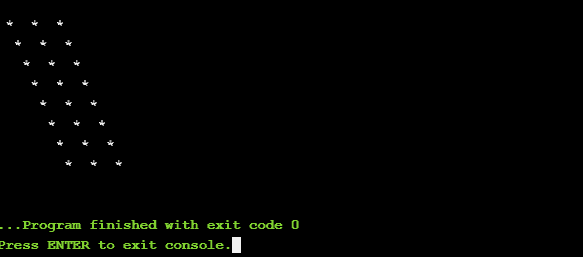CppBuzz.com

# C++ code to print * diagonally using while loop

This program uses three while loops to print * diagonally. Program can be done using other loops like for & do-while loops also.

``````#include <iostream>
using namespace std;

int main()
{
cout<<endl;
int i, j, ii;

i=8;
while(i>0){

ii=i;
while(ii<8){
cout<<" ";
ii++;
}

j=0;
while(j<3){
cout<<" * ";
j++;
}

i--;
cout<<endl;
}
return 0;
}
``````

## Printing * diagonally in C++